Levers Distance and velocity ratio. .Look at the lever on the right. The effort(piston) moves up 10cms while the end of the lever moves 30cms over the same time period. The distance and velocity ratios are therefore the same and can be calculated by the expression below.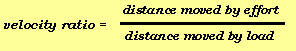In this case the velocity ratio is 1/3.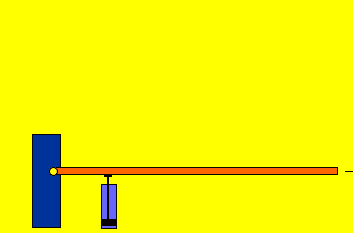You may have observed that the closer the effort is to the fulcrum the greater the distance the load moves through. Notice how little the lever is lifted by the piston on the right. One advantage, however, is the further away the effort is from the fulcrum the greater the load that can be lifted. The velocity ratio in this case is 1.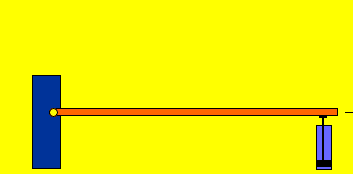Using levers to multiply the velocity of the load was used to great effect in medieval siege weapons. Huge boulders were hurled at castles with devastating effect.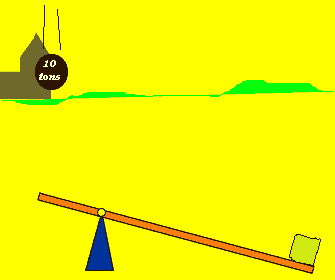What is one advantage and disadvantage of putting the hydraulic piston so close to the fulcrum?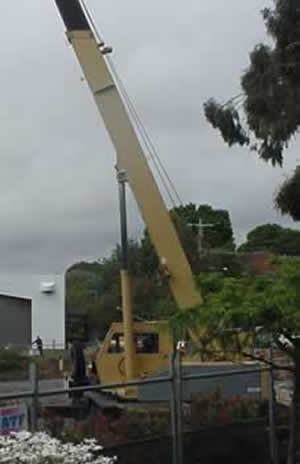Why is the biceps muscle connected to the forearm so close to the elbow joint?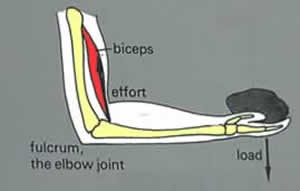Home